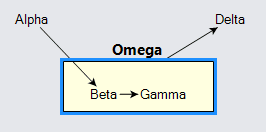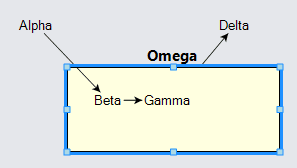# Groups Without Placeholders

Although it is very common to use a Placeholder inside a Group, it is not required. Using a Shape, for example, instead of a Placeholder permits features such as having a group maintain a fixed size, independent of the sizes and positions of its member nodes, and even when there are no member nodes at all. It also may allow the user to resize the "area" if that functionality is desired.

## Fixed size Groups

Not using a Placeholder in a Group means that you have to maintain the size and position of the group, because it cannot depend on the size and position of its member nodes. In these examples we will explicitly set and/or bind the Part.Location of the nodes, including the groups. The Shape that replaces the Placeholder in the group's template should also get its GraphObject.DesiredSize set or bound.

``````
diagram.NodeTemplate =
new Node()
.Bind("Location", "Loc", Point.Parse)

diagram.GroupTemplate =
new Group("Vertical") {
SelectionElementName = "PH",
LocationElementName = "PH"
}
.Bind("Location", "Loc", Point.Parse)
new TextBlock { Font = new Font("Segoe UI", 15, FontWeight.Bold) }  // group title
.Bind("Text", "Key"),
new Shape {  // using a Shape instead of a Placeholder
Name = "PH",
Fill = "lightyellow"
}
.Bind("DesiredSize", "Size", Size.Parse)
);

diagram.Model =
new MyModel {
NodeDataSource = new List<NodeData> {
new NodeData { Key = "Alpha", Loc = "0 0" },
new NodeData { Key = "Beta", Group = "Omega", Loc = "75 75" },
new NodeData { Key = "Gamma", Group = "Omega", Loc = "125 75" },
new NodeData { Key = "Omega", IsGroup = true, Loc = "50 50", Size = "150 50" },
new NodeData { Key = "Delta", Loc = "200 0" }
},
new LinkData { From = "Alpha", To = "Beta" },  // from outside the Group to inside it
new LinkData { From = "Beta", To = "Gamma" },  // this link is a member of the Group
new LinkData { From = "Omega", To = "Delta" }  // from the Group to a Node
}
};
diagram.Select(diagram.FindNodeForKey("Omega"));
``````Note that moving the "Beta" or "Gamma" nodes does not change the size or position of the "Omega" group. However moving or copying or deleting the group includes those member nodes in the operation.

One can control where the user may drag member nodes. For example, the Swim Lanes sample demonstrates a custom Part.DragComputation function that limits the motion of a member node to stay within its containing group.

## Resizable Groups

You can make the main shape resizable by the user. (At the current time groups are not rotatable.)

This example also makes the Part.Location and GraphObject.DesiredSize data bindings TwoWay, so that as the user moves groups or resizes their main shapes, the data in the model is updated automatically.

``````
diagram.NodeTemplate =
new Node()
.Bind("Location", "Loc", Point.Parse)

diagram.GroupTemplate =
new Group("Vertical") {
SelectionElementName = "PH",
LocationElementName = "PH",
Resizable = true,
ResizeElementName = "PH"
}
.Bind("Location", "Loc", Point.Parse, Point.Stringify)
new TextBlock { Font = new Font("Segoe UI", 15, FontWeight.Bold) }  // group title
.Bind("Text", "Key"),
new Shape {  // using a Shape instead of a Placeholder
Name = "PH",
Fill = "lightyellow"
}
.Bind("DesiredSize", "Size", Size.Parse, Size.Stringify)
);

diagram.Model =
new MyModel {
NodeDataSource = new List<NodeData> {
new NodeData { Key = "Alpha", Loc = "0 0" },
new NodeData { Key = "Beta", Group = "Omega", Loc = "75 75" },
new NodeData { Key = "Gamma", Group = "Omega", Loc = "125 75" },
new NodeData { Key = "Omega", IsGroup = true, Loc = "50 50", Size = "150 50" },
new NodeData { Key = "Delta", Loc = "200 0" }
},
new LinkData { From = "Alpha", To = "Beta" },  // from outside the Group to inside it
new LinkData { From = "Beta", To = "Gamma" },  // this link is a member of the Group
new LinkData { From = "Omega", To = "Delta" }  // from the Group to a Node
}
};
diagram.Select(diagram.FindNodeForKey("Omega"));
``````It is also possible to control how the user resizes a group. For example, the Swim Lanes sample demonstrates a custom ResizingTool that limits how small each lane can go. It also demonstrates a custom Adornment that has only two resize handles.

## Containers that are not Groups

You do not have to use Groups as the only mechanism by which to organize a collection of Parts. For example, the Layer Bands sample demonstrates how some Layouts can be customized to automatically maintain the positions and sizes of special parts that are in the background, appearing to surround the nodes that belong to each layout layer.

Not using Groups also means that it becomes possible to avoid some of the restrictions inherent in Groups, such as the limitation that each Part can have at most one Part.ContainingGroup. The Shared States sample demonstrates how one can make it appear that more than one "group" can contain a node. However, this requires some additional custom Tools and custom Layouts, or always explicitly setting/binding the location and size of every node and "group".